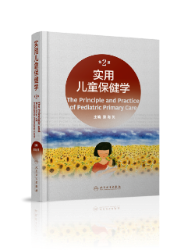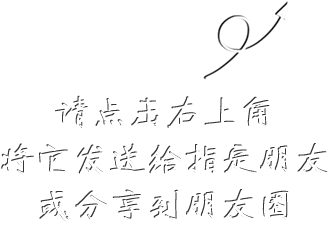999# 实用儿童保健学（第2版） 本书为预售，预计2022年6月30日后发货

## ¥ 278.40348.00现货已售出0件

• 内容全面、丰富

全书共7篇34章，在上一版基础上，补充新知识、新技术，从临床需要出发，全面讲述儿童保健临床实际内容。

• 基础理论与临床实践相结合

设立儿童保健学总论，体格生长与相关疾病，体格生长评价，神经、心理、行为发育与相关疾病，常见遗传性疾病诊断与鉴别，儿童营养、环境与健康，疾病预防等7篇，增加部分新章节，神经系统发育部分补充口腔运动敏感发育、大小便控制神经系统发育与功能鉴别，以及婴幼儿手势发育；内分泌常见疾病（甲状腺功能减退症与儿童糖尿病），功能性腹泻等；补充临床常见症状、体征鉴别，如斜头、枕秃、先天性降口角肌发育不良（歪嘴）、先天性束带综合征、扁平足、生长痛、舌系带短缩等；营养部分补充人乳喂养评估，人乳铁、维生素D、维生素K含量低的原因，婴儿期不宜食用蜂蜜，不必额外食用糖、盐，婴儿引入新食物时需避免发生高铁血红蛋白血症、胡萝卜血症等新内容，以飨读者。

• 适用人群广泛

丰富的知识量，引人入胜、页页精彩，读者都会有满满的收获，是儿科医生、儿内科医生、儿童保健医生不可多得的案头佳作。

• 删除
• 删除
• 删除
• 删除
• 删除
• 删除
• 删除
• 删除
• 删除
• 删除
• 删除
• 删除
• 删除
• 删除
• 删除
• 删除
• 删除
• 删除
• 删除
• 删除
• 删除
• 删除
• 删除
• 删除
• 删除
• 删除
• 删除
• 删除
• 删除
• 删除
• 删除
• 删除
• 删除
• 删除
• 删除
• 删除
• 删除
• 删除
• 删除
• 删除
• 删除
• 删除
• 删除
• 删除
• 删除
• 删除
• 删除
• 删除
• 删除
• 删除
• 删除
• 删除
• 删除
• 删除
• 删除
• 删除
• 删除
• 删除
• 删除
• 删除
• 删除
• 删除
• 删除
• 删除
• 删除
• 删除
• 删除
• 删除
• 删除
• 删除
• 删除
• 删除
• 删除
• 删除
• 删除
• 删除
• 删除
• 删除
• 删除
• 删除
• 删除
• 删除
• 删除
• 删除
• 删除
• 删除
• 删除
• 删除
• 删除
• 删除
• 删除
• 删除
• 删除
• 删除
• 删除
• 删除
• 删除
• 删除
• 删除
• 删除
• 删除
• 删除
• 删除
• 删除
• 删除
• 删除
• 删除
• 删除
• 删除
• 删除
• 删除
• 删除
• 删除
• 删除
• 删除
• 删除
• 删除
• 删除
• 删除
• 删除
• 删除
• 删除
• 删除
• 删除
• 删除
• 删除
• 删除
• 删除
• 删除
• 删除
• 删除
• 删除
• 删除
• 删除
• 删除
• 删除
• 删除
• 删除
• 删除
• 删除
• 删除
• 删除
• 删除
• 删除
• 删除
• 删除
• 删除
• 删除
• 删除
• 删除
• 删除
• 删除
• 删除
• 删除
• 删除
• 删除
• 删除